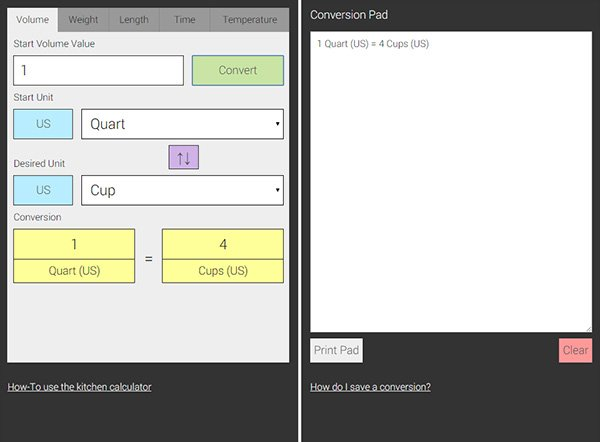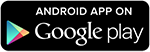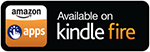## KITCHEN CALCULATOR

The kitchen calculator (also known as a kitchen converter, kitchen unit converter, baking unit converter, or cooking unit converter) is a kitchen tool that can be used to help you convert the most common units used in cooking and baking. The kitchen calculator tool also has a conversion pad that helps you keep track of recent conversions and allows you to edit or add notes.

See the instructions below or visit the How-To section for details on how to use the kitchen calculator.

You can also download the MyKitchenCalculator.com App (currently only available on Android and Kindle devices), which includes most of the conversion tools found on our website and additional tools such as an ingredient unit converter that allows you to convert between volume and weight measurements for more then 250 ingredients!

Harness the power of recipe conversion!
(Not available for iOS devices.)

Start Volume Value
Start Unit
Desired Unit

Conversion
=
Start Weight Value
Start Unit
Desired Unit

Conversion
=
Start Length Value
Start Unit
Desired Unit

Conversion
=
Start Time Value
Start Unit
Desired Unit

Conversion
=
Start Temperature Value
Start Unit
Desired Unit

Conversion
=
Gas Mark
=
OR

## How to use the Kitchen Calculator

(Kitchen Converter / Kitchen Unit Converter / Baking Unit Converter / Cooking Unit Converter / Recipe Unit Converter)Volume Unit Converter:

The volume unit converter allows you to convert between the most common volume units used in cooking and baking. Some of these units include: teaspoons, tablespoons, cups, pints, quarts, gallons, fluid ounces, milliliters, and liters.

1. Enter a starting value between 0 and 1,000,000.

2. Press the start unit US/UK button to toggle between a start US or UK unit, and select a start unit.

3. Press the desired unit US/UK button to toggle between a desired US or UK unit, and select a desired unit.

4. Press the convert button.

5. The unit conversion will appear in the conversion area.

Weight Unit Converter:

The weight unit converter allows you to convert between the most common weight units used in cooking and baking. Some of these units include: grams, kilograms, ounces, and pounds.

1. Enter a starting value between 0 and 1,000,000.

2. Choose a start unit and a desired unit.

3. Press the convert button.

4. The unit conversion will appear in the conversion area.

Length Unit Converter:

The length unit converter allows you to convert between the most common length units. Some of these units include: millimeters, centimeters, meters, kilometers, inch, feet, yards, and miles.

1. Enter a starting value between 0 and 1,000,000.

2. Choose a start unit and a desired unit.

3. Press the convert button.

4. The unit conversion will appear in the conversion area.

Time Unit Converter:

The time unit converter allows you to convert between the most common time units. Some of these units include: seconds, minutes, hours, days, months, and years.

*(The month unit is based on approximately 30.42 days and the year unit is based on 365 days)

1. Enter a starting value between 0 and 1,000,000.

2. Choose a start unit and a desired unit.

3. Press the convert button.

4. The unit conversion will appear in the conversion area.

Temperature Unit Converter:

The temperature unit converter allows you to convert between the most common temperature units. Some of these units include: Celsius, Fahrenheit, and Kelvin. The temperature converter also displays Gas Mark temperature references.

Temperature:

1. Enter a starting value between -1,000 and 1,000.

2. Choose a start unit and a desired unit.

3. Press the convert button.

4. The unit conversion will appear in the conversion area.

Gas Mark:

1. Select a Gas Mark number from the dropdown selection box.

2. The temperature reference will appear in the conversion area.

1. The conversion pad text area will display a history of the most recent unit conversions. The contents of the conversion pad can be edited, printed, or copied and pasted into a document.

2. Fractions can be entered as a value. When using fractions, separate whole numbers from fractions with a space.

examples:
One-third = "1/3"
One and one-half = "1 1/2".

3. Volume conversions are based on rounded values and are not exact. This converter may not be suitable for engineering, scientific, or mathematical purposes.

4. The year unit is based on a standard 365 day period. The month unit is based on approximately 30.42 days.